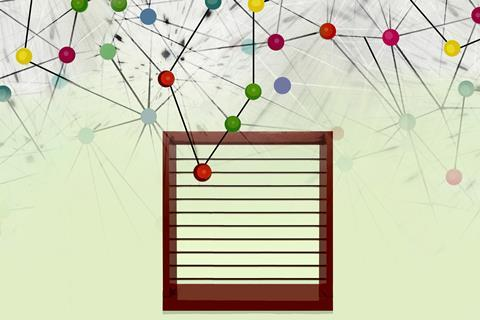Why students can get the right answers without understanding the conceptsPictorial questions may be a better diagnostic tool than numerical questions for measuring students’ chemical kinetic understanding

A study has shown that problems requiring interpretation of diagrams or graphs are useful for measuring students’ understanding of chemical kinetics. The research also revealed differences in the way students approach algorithmic and pictorial problems.

Chemical kinetics is a difficult topic for many students. They need a good understanding of the underlying concepts and a firm grasp of mathematics.

Students’ understanding of kinetics is mainly assessed through numerical problems. However, good performance can mask poor conceptual understanding. This is because numerical problems are algorithmic. This means that, generally, students need to manipulate a formula to compute a numerical answer using a plug-and-chug method, and they can solve the problems without fully understanding the underlying concepts. This contrasts with conceptual problems, which are often pictorial in nature and require the interpretation of a diagram or graph.

## Kinetic misunderstanding

The research team, from Indonesia and the UK,  examined student performance variations in a series of paired problems in chemical kinetics. 335 first-year undergraduate chemistry students in Indonesia and the UK participated.

One question in each problem pair was algorithmic, the other pictorial. Each pair of problems examined the same fundamental concept, but presented information differently and required different information processing and analytical steps. For example, one problem pair examined the impact of students’ understanding of reactant order upon reaction rate. The algorithmic problem involved typical handling of reaction rate and reactant concentration data, using given reaction orders, to determine the rate equation. These kinds of question are seen in many post-16 curriculums. The pictorial problem encoded this information in microscopic particle diagrams.

The results showed that the students’ performance in algorithmic problems was generally higher than in the pictorial problems. For example, students were able to select and use appropriate equations to get the correct answer in the algorithmic problem relating to the concept of half-life. However, the pictorial problem revealed a poor understanding of the concept of half-life. Therefore, pictorial questions may be a better diagnostic tool for dealing with misconceptions.

Also, students’ incorrect answers were different for the algorithmic problems compared to the pictorial ones, suggesting that students approached the question types differently.

## Teaching tips

• You can access the test instrument that was used in this study for free. Try it in your classroom.
• For chemistry topics with a large mathematical component, good student performance on algorithmic problems does not necessarily mean they have understood the underlying concepts well.
• Algorithmic problems test students’ ability to select the correct mathematical formula and use it to get a numerical answer, while more conceptual problems test students’ ability to make links between the various representations used in chemistry (ie Johnstone’s triangle).
• Use pictorial problems as a diagnostic tool to address misconceptions you may not otherwise have been aware of.